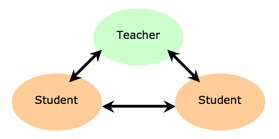Te Kete Ipurangi
Communities
Schools

# Formative assessment occurs DURING learning

## What this looks like in mathematics and statistics

Formative assessment enables a teacher to:

• provide students with feedback to improve and enhance learning
• understand student learning
• build a picture of student progress
• inform planning decisions, may involve further diagnosis
• adapt teaching to meet student learning needs.Formative assessment

Questions teachers and students should be asking:

• What are we trying to do?
• How well have we done it?
• How do we know?
• What do we do when we don’t know what to do?

### Specific activities

• Students posing and answering questions.
• Students evaluating questions in a textbook exercise to decide which ones they should work on.
• Asking 'What is the same and what is different?', for example, for linear or quadratic functions.
• Asking students to 'find another example . . . and another', for example, a function has x-intercepts 3 and 5.
• Giving an A5 piece of scrap paper for students to record their thinking about what they learned, need to know, would like help with.
• Exit slips: Exit slips are a quick assessment tool for teachers to use as part of their daily classroom routine.
1. The teacher poses a question for the students to respond to. The question must be short and shouldn’t take students long to complete.
2. Students complete on a slip of paper or card.
3. As the students leave the classroom they hand in their exit slip.
4. The classroom teacher now has a quick assessment tool. By assessing the responses on the exit slips the teacher can better differentiate the instruction in order to accommodate students' needs for next lesson.
• Examples of exit slip questions:
• write one thing you learned today
• write one question you have about today's lesson
• of the 3 graphs we studied today, which one did you find most useful? Why?
• Using cooperative activities, for example, Get it Together, EQUALS.
• Using counter-examples to disprove conjectures, for example, every quadratic graph cuts the x-axis twice.
• Giving the answer and asking what is the question, for example, a quadratic equation has solutions ½ and 3, what is the equation?
• Self-assessment
• Peer-assessment
• Homework
• Lesson diagnostic snapshot, for example, ask the students questions such as: 'Find fractions between these pairs of fractions; and , and..'.
• Use of mini-whiteboards for students to show their answer to a question.
• Quiz at beginning of a lesson.
• Student generated test or practice examples.

### Further information

• Erickson, T. (1989). Get it Together: Maths Problems for Groups.
A resource for problem solving in groups where each member has a clue but cannot show it to the rest of the group.
• William, D. (2007). Ahead of the Curve: The Power of Assessment to Transform Teaching and Learning. Content then process: Teacher learning communities in the service of formative assessment.

Last updated September 17, 2018